# Delta Sigma Toolbox

Idin's pick for this week is the Delta Sigma Toolbox by Richard Schreier.

Delta-Sigma (or sigma-delta) modulators are commonly found in electronic components such as analog-to-digital and digital-to-analog converters (ADCs and DACs), and increasingly in [fractional-N] frequency synthesizers (PLLs), and switch-mode power supplies. Generally speaking, a delta-sigma modulator produces a highly over-sampled binary signal at its output (0/1) which can be low-pass filtered to reproduce the input signal. Figure 1 below shows an example input & output signal. To read more about delta-sigma modulators, go here, here, and here. Richard’s textbook is also a good resource: "Understanding Delta-Sigma Data Converters" by Schreier and Temes (ISBN 0-471-46585-2).

There is a large body of literature dedicated to analysis and design of delta-sigma modulators, and the Delta Sigma Toolbox provides a great tool for analyzing these components in MATLAB. The toolbox contains a fairly complete list of well documented functions to construct, analyze, and simulate delta-sigma modulators of arbitrary order. The full documentation for the toolbox is provided in DSToolbox.pdf (part of the download).

To start using the toolbox, I highly recommend studying the provided example and demo files (dsdemo1-8.m and dsexample1-4.m). I like dsdemo2.m as a starting tutorial. It begins by creating a noise transfer function (NTF), and then simulates the modulator with an example sinusoidal input:

OSR = 32;
H = synthesizeNTF(5,OSR,1);
N = 8192;
fB = ceil(N/(2*OSR)); ftest=floor(2/3*fB);
u = 0.5*sin(2*pi*ftest/N*[0:N-1]);  % half-scale sine-wave input
v = simulateDSM(u,H);


The time domain output shows the sinusoidal input (red) and the binary output (green).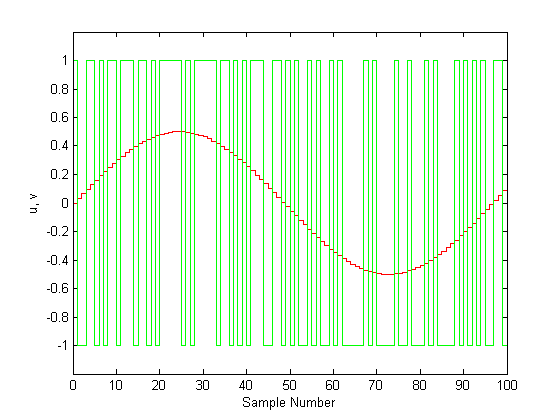The spectrum of the output can also be easily computed using MATLAB's FFT function: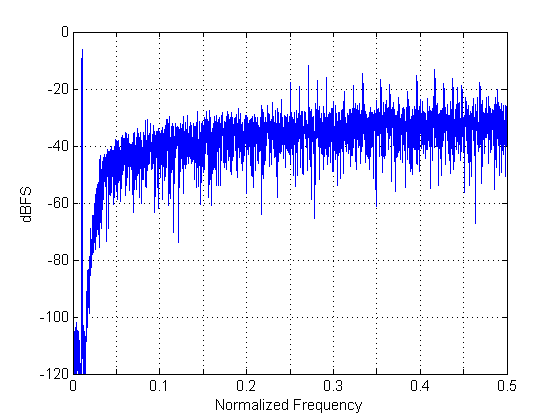This figure looks like the superposition of two signals: the spike at ftest/N (0.0104 in this case) which represents the input sinusoid, and some high frequency "noise". The high-pass noise is in fact one of the desirable properties of delta-sigma converters; these converters shift the quantization noise to high frequencies, i.e., away from our signal/carrier of interest. It should be self-evident from Figure 2 that a simple low-pass filter of this output signal would yield the original sinusoidal input.

The next few lines in dsdemo2.m compute the SNR on the output signal, and also use Delta Sigma Toolbox functions to compute and display the expected (theoretical) response of the modulator (shown in pink blow).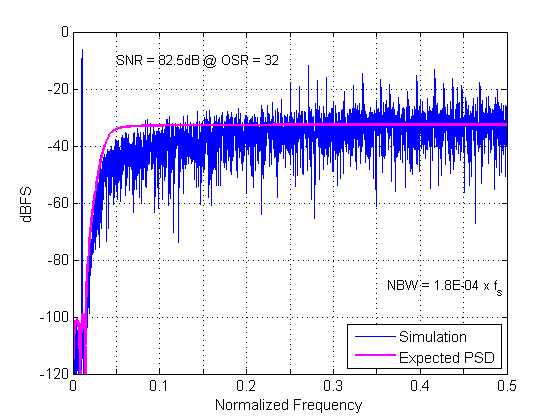The next section of the code computes the expected and simulated signal-to-quantization-noise ( SQNR), which is a figure of merit when analyzing analog-to-digital converters.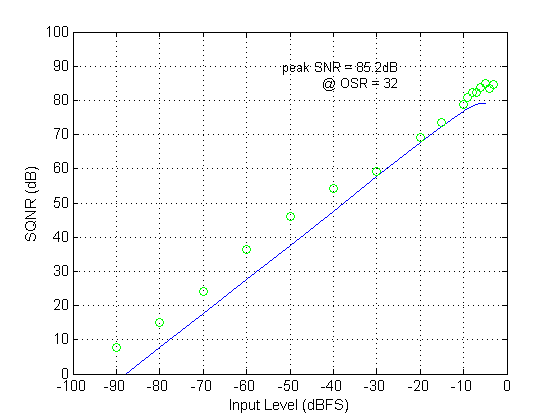The remainder of this demo essentially repeats the same process, but for a band-pass signal. That is, instead of using a low frequency sinusoid as the input, it uses a carrier signal, which makes our output spectrum look as in Figure 5 (the carrier here is at Fs/8 or 0.125 on the normalized frequency axis).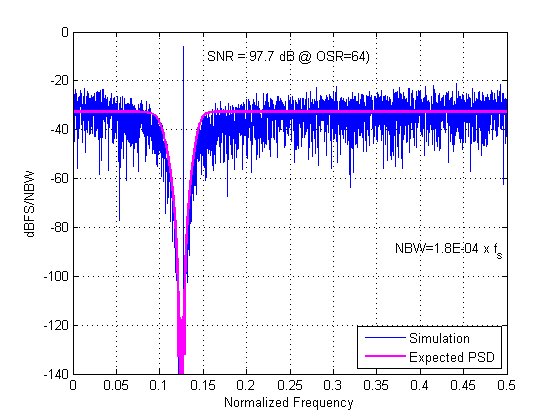I encourage you to download the toolbox and run through the demos. Even if you do not use the toolbox for any simulations of your own, these demos can serve as a great learning tool (along with freely available online resources mentioned above).

Other MathWorks resources:

If you are interested in delta-sigma modulators (and other mixed-signal components), consider downloading the Mixed-Signal Library for Simulink. Also, take a look at the MathWorks Mixed-Signal page, particularly the ADC section.

Delta-Sigma Toolbox Usage notes:

• A few C files are provided in the Toolbox that the user needs to compile to MEX (e.g., simulateDSM.c). This is generally done as:
mex simulateDSM.c


But as noted in the comments on File Exchange, this fails on some systems. Simply defining the _STDC_ symbol should resolve the issue:

mex simulateDSM.c –D__STDC__


Note that you will need a C compiler for this step. Run mex –setup at the MATLAB command prompt to setup your C compiler.

• If you plan on using the Delta Sigma Toolbox frequently, you should probably add the toolbox folder to your MATLAB path:
addpath('<your_intall_path>\delsig')
savepath


Suggestions for improvements

• A great addition to the provided example/demo files would be the use of sections, and perhaps the publish feature to include a full write-up and description for each file.
• Providing an Install script would be great (to take care of MATLAB path and compiling MEX files).